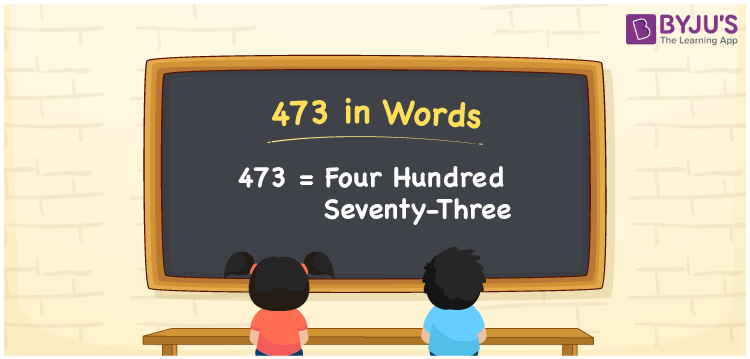# 473 in Words

The numeral 473 in English words is “four hundred seventy-three”. If you spent Rs. 473 on purchasing flowers, you can write, “I spent Rs. Four hundred seventy-three on purchasing flowers”. Also, we can say that the number 473 is a cardinal number

 473 in Words: Four Hundred Seventy-three. Four Hundred Seventy-three in Numerical Form: 473.

## 473 in English Words## How to Write 473 in Words?

The place value table for the number 473 is presented below:

 Hundreds Tens Ones 4 7 3

The expanded form of 473 is as follows:

= 4 × Hundred + 7 × Ten + 3 × One

= 4 × 100 + 7 × 10 + 3 × 1

= 400 + 70 + 3

= 473

= Four hundred seventy-three

Hence, 473 in words is four hundred seventy-three.

473 in words – Four hundred seventy-three

Is 473 an odd number? – Yes

Is 473 an even number? – No

Is 473 a perfect square number? – No

Is 473 a perfect cube number? – No

Is 473 a prime number? – No

Is 473 a composite number? – Yes

## Frequently Asked Questions on 473 in Words

Q1

### Write 473 in words.

473 in words is four hundred seventy-three.

Q2

### Simplify 400 + 73, and express it in words.

Simplifying 400 + 73, we get 473. Hence, 473 in words is four hundred seventy-three.

Q3

### Is 473 an odd number?

Yes, 473 is an odd number.